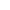Maths | ICSE Board | Grade 12 | 2016
Q. Using properties of definite integrals, evaluate:
π20sinxcosx1+sinxcosxdx
View SolutionQ. Find the area of the region bound by the curves y=6xx2 and y=x22x.
View SolutionQ. The two lines of regressions are x+2y5=0 and 2x+3y8=0 and the variance of x is 12. Find the variance of y and the coefficient of correlation.
View SolutionQ. An urn contains 10 white and 3 black balls while another urn contains 3 white and 5 black balls. Two balls are drawn from the first urn and put into the second urn then a ball is drawn from the latter. Find the probability that it is a white ball.
View SolutionQ. Evaluate: tan3xdx
View SolutionQ. Find the Cartesian equation of the plane, passing through the line of intersection of the planes: r.(2^i+3^j4^k)+5=0 and r.(^i5^j+7^k)+2=0 and intersecting y-axis at (0, 3)
View SolutionQ. Calculate Karl Pearson's coefficient of correlation between x and y for the following data and interpret the result:
(1, 6), (2, 5), (3, 7), (4, 9), (5, 8), (6, 10), (7, 11), (8, 13), (9, 12)
View SolutionQ. A company manufactures two types of products A and B. Each unit of A requires 3 grams of nickel and 1 gram of chromium, while each unit of B requires 1 gram of nickel and 2 grams of chromium. The firm can produce 9 grams of nickel and 8 grams of chromium. The profit is Rs. 40 on each unit of product of type A and Rs. 50 on each unit of type B. How many units of each type should the company manufacture so as to earn maximum profit? Use linear programming to find the solution
View SolutionQ. A pair of dice is thrown. What is the probability of getting an even number on the first die or a total of 8?
View SolutionQ. Find the equation of the standard ellipse, taking its axes as the coordinate axes, whose minor axis is equal to the distance between the foci and whose length of latus rectum is 10. Also, find its eccentricity.
View SolutionQ. Solve for x, if
tan(cos1x)=25
View SolutionQ. A rectangle is inscribed in a semicircle of radius r with one of its sides on the diameter of the semicircle. Find the dimensions of the rectangle to get maximum area. Also, find the maximum area.
View SolutionQ. Find the locus of a complex number, z=x+iy, satisfying the relation z3iz+3i2
Illustrate the locus of z in the Argand plane.
View SolutionQ. Express 2+i(1+i)(12i) in the form of a+ib. Find its modulus and argument.
View SolutionQ. A bill of Rs. 1800 drawn on 10th September, 2010 at 6 months was discounted for Rs. 1782 at a bank. If the rate of interest was 5% per annum, on what date was the bill discounted.
View SolutionQ. Solve the following differential equation:
x2dy+(xy+y2)dx=0, when x=1 and y=1
View SolutionQ. A committee of 4 persons has to be chosen from 8 boys and 6 girls, consisting of at least one girl. Find the probability that the committee consists of more girls than boys.
View SolutionQ. Write the Boolean function corresponding to the switching circuit given below:View SolutionQ. Solve the differential equation:
xdydx+y=3x22
View SolutionQ. Evaluate: sinx+cosx9+16sin2xdx
View SolutionQ. The demand function is x=242p3 where x is the number of units demanded and p is the price per unit. Find:
(i) The revenue function R in terms of p.
(ii) The price and the number of units demanded for which the revenue is maximum.
View SolutionQ. The marks obtained by 10 candidates in English and Mathematics are given below:
 Marks in English 20 13 18 21 11 12 17 14 19 15 Marks in Mathematics 17 12 23 25 14 8 19 21 22 19
Estimate the probable score for Mathematics if the marks obtained in English are 24.
View SolutionQ. The difference between mean and variance of a binomial distribution is 1 and the difference of their squares is 11. Find the distribution.
View SolutionQ. Find the image of the point (2, 1, 5) in the line x1110=y+24=z+811
Also, find the length of the perpendicular from the point (2, 1, 5) to the line.
View SolutionQ. In an automobile factory, certain parts are to be fixed into the chassis in a section before it moves into another section. On a given day, one of the three persons A, B and C carries out this task. A has 45% chance, B has 35% chance and C has 20% chance of doing the task. The probability that A, B and C will take more than the allotted time is 16, 110 and 120 respectively. If it is found that the time taken is more than the allotted time, what is the probability that A has done the task?
View SolutionQ. Find a unit vector perpendicular to each of the vectors a+b and ab where a=3^i+2^j+2^k and b=^i+2^j2^k.
View SolutionQ. Using L' Hospital rule, evaluate: limx0(1x2cotxx)
View SolutionQ. Find the matrix for which:
X=
View SolutionQ. A man borrows Rs. 20, 000 at 12% per annum, compounded semi-annually and agrees to pay it in 10 equal semi-annual installments. Find the value of each installment, if the first payment is due at the end of two years.
View SolutionQ. Solve the following system of linear equations using matrix method:
3x+y+z=1, 2x+2z=0, 5x+y+2z=2
View Solution